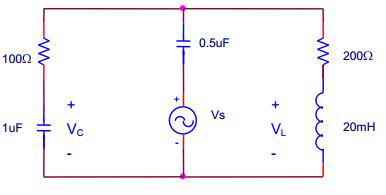# RLC Circuit total capacitance

• Engineering
hogrampage

## Homework Statement

Find VL(t) and Vc(t) in the circuit belowWhere Vs = cos(200$\pi$t) V

## Homework Equations

$\alpha$ = $\frac{1}{2RC}$
ω0 = $\frac{1}{\sqrt{LC}}$
$\omega$0 = $\sqrt{\omega^{2}-\alpha^{2}}$

## The Attempt at a Solution

Well, I found the total capacitance

C = 1.5 uF

Then, I found $\alpha$:

$\alpha$ = $\frac{1}{2(100Ω)(1.5uF)}$ ≈ 3333 s-1

and

ω0 = $\frac{1}{\sqrt{(20mH)(1.5uF)}}$ ≈ 5774 s-1

So Vc = B1e-3333cos($5774\pi$t) + B2e-3333sin($5774\pi$t)

That's as far as I went. I have no idea what I'm doing :|. Also, please note that I have NOT had differential equations. This class does not require it, so please give an answer without using DQ's.

## Answers and Replies

hogrampage
I looked through some articles/lessons on these types of circuits, and they used impedance to solve for voltage drops and all that. However, none of them showed a time-dependent function. So, can I solve this problem by using similar steps (i.e. find XL, XC, and then impedance Z)?

Here are the values I came up with (noting that XC is inductive reactance of both capacitors combined):

XL = 2$\pi$(200$\pi$)(20mH) = 78.96Ω
XC = 1/2(200$\pi$)(1.5uF) = 168.87Ω
Z = 1/(sqrt((1/R)2+(1/XL-1/XC)^2) = 81.56Ω
I = V/Z = 1/81.56 = 10mA

Homework Helper
Gold Member
No picture.

Antiphon
Have you learned to use phasors yet? That would make the problem look like it was all resistors with complex numbers (phasors) for voltage and current.

hogrampage
Unfortunately, no. We are starting the chapter with those tomorrow. This assignment is supposed to use the form that looks something like this:

A-Ae^-t/tau

Homework Helper
Gold Member
I looked through some articles/lessons on these types of circuits, and they used impedance to solve for voltage drops and all that. However, none of them showed a time-dependent function. So, can I solve this problem by using similar steps (i.e. find XL, XC, and then impedance Z)?

Here are the values I came up with (noting that XC is inductive reactance of both capacitors combined):

XL = 2$\pi$(200$\pi$)(20mH) = 78.96Ω
XC = 1/2(200$\pi$)(1.5uF) = 168.87Ω
Z = 1/(sqrt((1/R)2+(1/XL-1/XC)^2) = 81.56Ω
I = V/Z = 1/81.56 = 10mA

No, because that does not account for the phasing of the voltages within your circuit. Like Antiphon says, you need to invoke phasors (comlex values for impedances, voiltages and currents). Pay particular attention to the fact that your voltage source is a cosine rather than the more usual sine generator.

Homework Helper
Gold Member
I looked through some articles/lessons on these types of circuits, and they used impedance to solve for voltage drops and all that. However, none of them showed a time-dependent function. So, can I solve this problem by using similar steps (i.e. find XL, XC, and then impedance Z)?

No, because that does not consider phasing of voltages and currents. Like Antiphon says, you need to invoke phasors (complex V, I and X). Also take note in this regard that your voltage source is a cosine rather than the more usual sine generator.

hogrampage
Okay, that makes sense. However, at the time of this (it was a lab yesterday), we had not learned about phasors, so I'm not sure how the professor wants us to do it. I do know I can use this as the voltage source:

sin(200$\pi$t+$\frac{\pi}{2}$)

So now that I got that, I'm not sure where to go. This problem was for the pre-lab, so I'm wondering how to solve it without phasors. It doesn't seem possible.

Homework Helper
Gold Member
Unfortunately, no. We are starting the chapter with those tomorrow. This assignment is supposed to use the form that looks something like this:

A-Ae^-t/tau

That's not what your answer will look like. You have a sinusoidal excitation plus you have BOTH inductor & capacitor. Either fact precludes a response like you state and in fact necssitates a sinusoidal one for both Vl and VC..

If this is doable without either phasors, diff. eq.'s or a transform method, I don't know what it would be but I do know I wouldn't want to find out, since for sure it would be a very awkward process. It reminds me of the ARRL Handbook, trying to teach this stuff without the necessary tools. I would sit on it until you get the phasor stuff given to you.